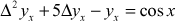## Difference Equations

##### 11.0 INTRODUCTION

Difference equations may be considered as the discrete analogue of differential equations. Discrete functions occur in many physical and engineering problems. So, difference equations are the natural choice for dealing with discrete situations.

Basic concepts of difference equations are similar to differential equations.

Definition 11.1: Difference equation

A difference equation is an equation involving an independent variable x, dependent variable yx and the successive differences of yx or successive values yx, yx+1, yx+2 ...

1. Example (1)2. are difference equations.

Since , every difference equation ...

Get Numerical Analysis, 1/e now with the O’Reilly learning platform.

O’Reilly members experience books, live events, courses curated by job role, and more from O’Reilly and nearly 200 top publishers.